Clinic Data Clinic Data is to add your Clinic Information. This Clinic Information will come in the top of the Invoice. Adding Clinic Information: Select Clinic Data from Master Menu. Add Clinic Name and other details in the appropriate columns in Clinic Data Tab .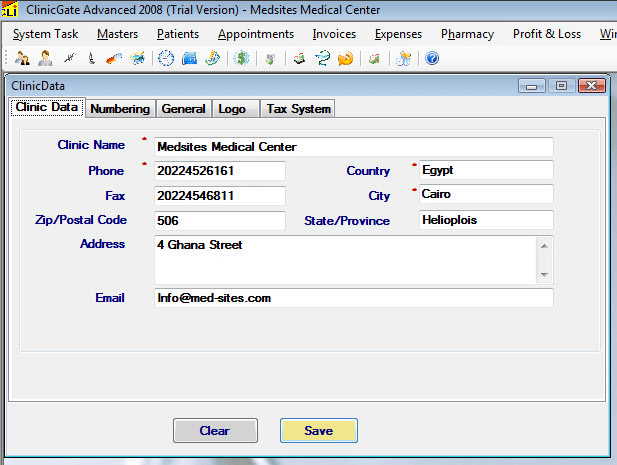In Numbering Tab: Patient File Number is to generate the File # in Patient File. Auto Number will generate the serial number automatically. Auto Number with Letters will generate the serial number with the preceding the letters given Manual will allows the user to key in the File #. Invoice File Number is to generate the Invoice # in the Invoice. Auto Number will generate the serial number automatically. Auto Number with Letters will generate the serial number with the preceding the letters given Manual will allows the user to key in the Invoice #, and the same for Company File Number, Charges File Number and Purchase File Number .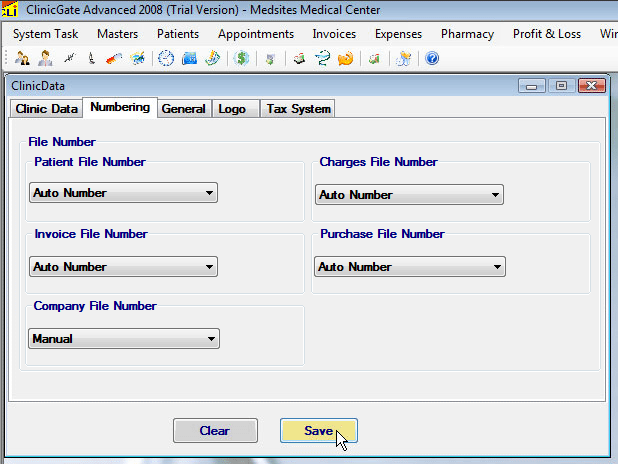In General Tab: You can find Date format , Base Currency & Financial year. Also you can add new currency by click Add Icon to open pop up window and write the currency and its symbol then click add in pop up window Cancel button in pop up window To clear Currency and symbol fields to add new one Delete currency by select it from Base Currency list and click delete Icon.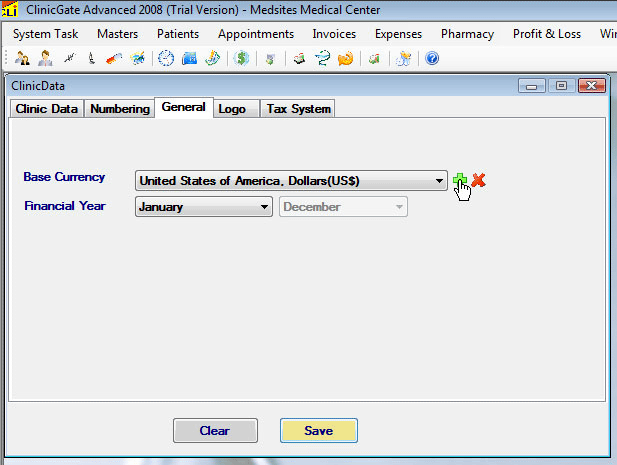Logo Tab: you can upload the logo of your Clinic with dimension(width 160 px, height 68 px).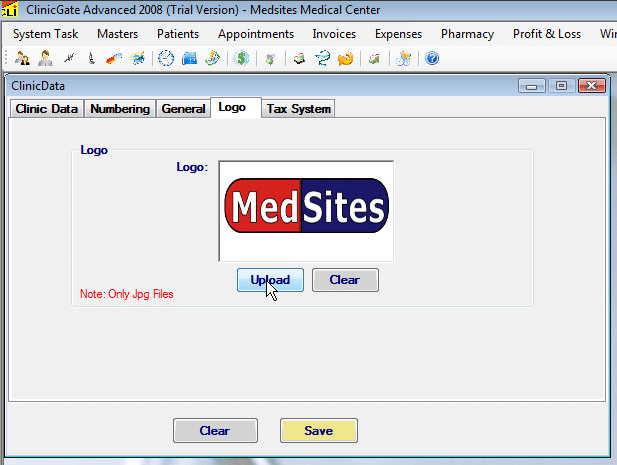In Tax System Tab : To add Sales Taxes and its Alias ,Also Select Tax Formula.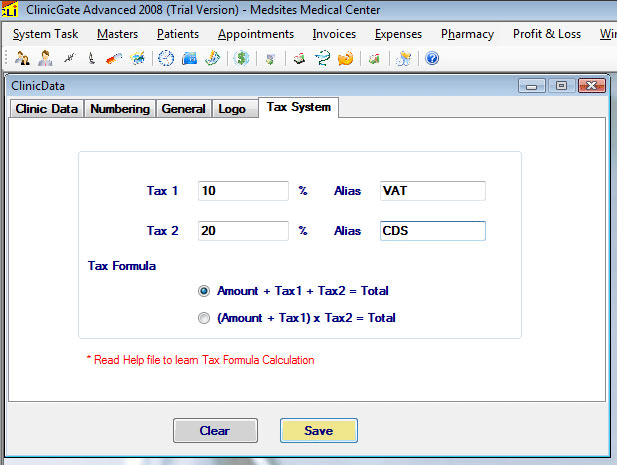If We have Amount=100 , Tax 1=10% , Tax 2=20% *And select the Formula [Amount + Tax 1+Tax 2=Total],then The Following Example Illustrate How the Tax Formula Calculation: the Tax 1 = (Amount/100)x10 = (100/100)x10 = 10 the Tax 2 = (Amount/100)x20 = (100/100)x20 = 20 Total after Tax 1 = Amount+Tax1=100+10 = 110 Total after Tax 2 = Total after Tax 1+Tax2=110+20 = 130 Total =130 *And If select the Formula [(Amount + Tax 1)xTax 2=Total] , then The Following Example Illustrate How the Tax Formula Calculation: the Tax 1 = (Amount/100)x10 = (100/100)x10 = 10 Total after Tax 1=Amount+Tax1 = 100+10 = 110 the Tax 2 = [(Total after Tax 1)/100]x20 = (110/100)x20 = 22 Total after Tax 2=110+22=132 Total =132 Editing Clinic Information You can edit Clinic information by writing the information you want and then click Save Button. Clear button To Clear the Clinic Data fields for adding new one by click Clear.

–>